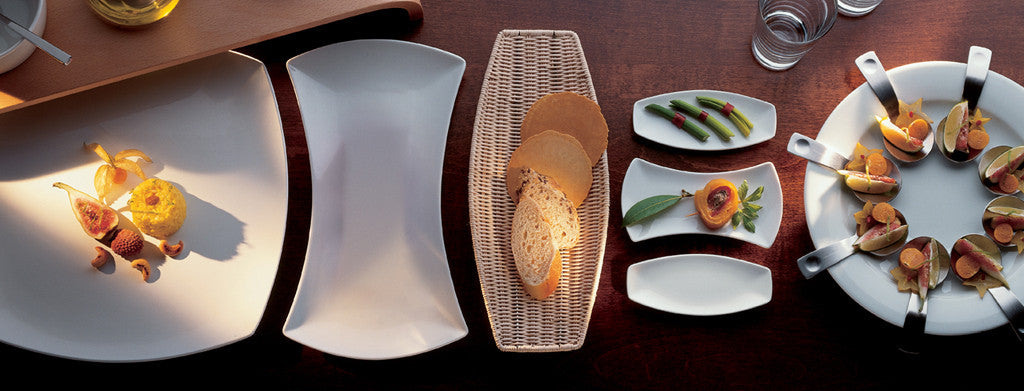Shopping Cart

### Refine

View all

#### Price# Crockery£2.66 each (ex VAT)£4.18 each (ex VAT)£3.08 each (ex VAT)£12.31 each (ex VAT)£9.88 each (ex VAT)£8.39 each (ex VAT)£8.57 each (ex VAT)£7.89 each (ex VAT)£5.75 each (ex VAT)£4.92 each (ex VAT)£3.99 each (ex VAT)£5.29 each (ex VAT)£4.73 each (ex VAT)£3.45 each (ex VAT)£6.00 each (ex VAT)£3.51 each (ex VAT)£16.28 each (ex VAT)£18.47 each (ex VAT)£10.13 each (ex VAT)£9.45 each (ex VAT)£8.43 each (ex VAT)£2.70 each (ex VAT)£4.42 each (ex VAT)£7.15 each (ex VAT)£3.41 each (ex VAT)£3.88 each (ex VAT)£3.61 each (ex VAT)£1.99 each (ex VAT)£3.89 each (ex VAT)£3.36 each (ex VAT)£3.72 each (ex VAT)£5.29 each (ex VAT)£5.48 each (ex VAT)£23.65 each (ex VAT)£3.09 each (ex VAT)£3.98 each (ex VAT)£5.82 each (ex VAT)£8.61 each (ex VAT)£13.81 each (ex VAT)£9.53 each (ex VAT)£7.25 each (ex VAT)£14.40 each (ex VAT)£10.85 each (ex VAT)£4.91 each (ex VAT)£7.72 each (ex VAT)